Profilers > Profiler > Additional Examples of the Prediction Profiler > Example of a Noise Factor in the Prediction Profiler
Publication date: 04/12/2021

## Example of a Noise Factor in the Prediction Profiler

This example uses the Tiretread.jmp sample data set. This data set shows the results of a tire manufacturer’s experiment whose objective is to match a target value of HARDNESS= 70 based on three factors: SILICA, SILANE, and SULFUR content. Suppose the SILANE and SULFUR content are easily (and precisely) controllable, but SILICA is not easily controlled.

For comparison, first optimize the factors for hardness without considering variation from the noise factor, SILICA.

1. Select Graph > Profiler to launch the Prediction Profiler.

2. Assign Pred Formula HARDNESS to the Y, Prediction Formula role.

3. Click OK.

4. Click the Prediction Profiler red triangle and select Optimization and Desirability > Desirability Functions.

5. Click the Prediction Profiler red triangle and select Optimization and Desirability > Set Desirabilities to open the Response Goal window.

Tip: Alternatively, you can double-click in the Desirability plot to open the Response Goal window.

6. Select Match Target from the list and click OK.

7. Click the Prediction Profiler red triangle and select Optimization and Desirability > Maximize Desirability to find the optimum factor settings for the target value of HARDNESS.

We get the following Prediction Profiler display. Notice that the SILICA factor’s optimum value is on a sloped part of a profile curve. This means that variations in SILICA settings impact the response, HARDNESS.

Note: You might get different results from these because different combinations of factor values can all hit the target.

Figure 3.20 Maximizing Desirability for HARDNESS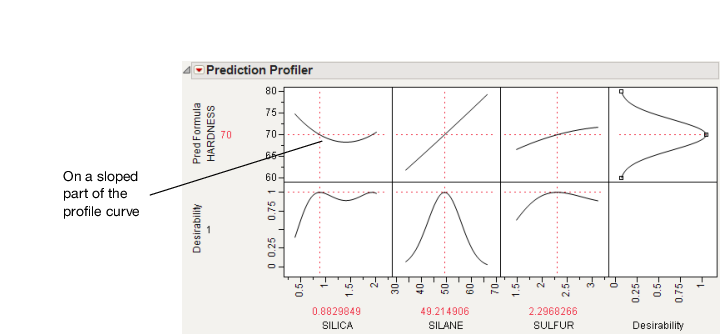Now, we would like to not just optimize for a specific target value of HARDNESS, but also find a setting that is insensitive to the variability in the setting of Silica. Repeat the optimization process with SILICA as a noise factor.

1. Select Graph > Profiler.

2. Select Pred Formula HARDNESS and click Y, Prediction Formula.

3. Select SILICA and click Noise Factors.

4. Click OK.

5. Select Optimization and Desirability > Desirability Functions in the Prediction Profiler menu.

6. Double-click in the Desirability plot for the response Pred Formula HARDNESS to open the Response Goal window.

7. Select Match Target from the list and click OK.

The resulting profiler has the appropriate derivative of the fitted model with respect to the noise factor, set to be maximized at zero, its flattest point.

Figure 3.21 Derivative of the Prediction Formula with Respect to Silica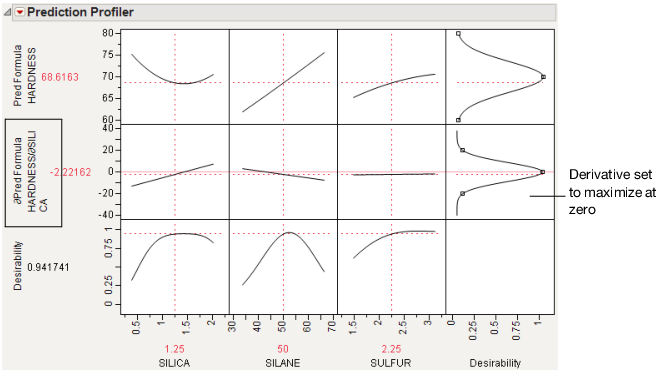8. Click the Prediction Profiler red triangle and select Optimization and Desirability > Maximize Desirability to find the optimum values for the process factor, balancing for the noise factor.

This time, we have also hit the targeted value of HARDNESS, but our value of SILICA is on its flatter region. This means variation in SILICA does not transmit as much variation to HARDNESS as when it was set at a value on the steep part of the SILICA curve.

Figure 3.22 Maximize Desirability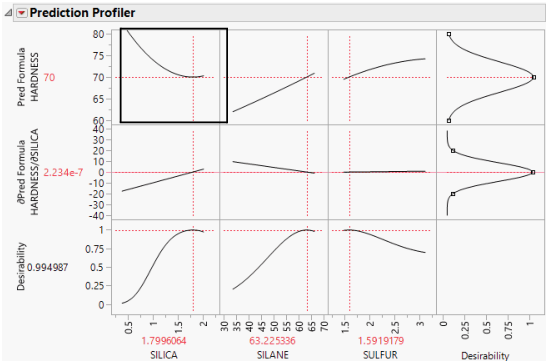You can see the effect of accounting for the SILICA variation on the variance of the predictions by following these steps for each profiler (one without the noise factor, and one with the noise factor):

1. In the Profiler without the noise factor, click the Prediction Profiler red triangle and select Simulator.

2. Assign SILICA to have a random Normal distribution with a standard deviation of 0.05. Use the default setting for the mean.

Figure 3.23 Setting a Random Normal Distribution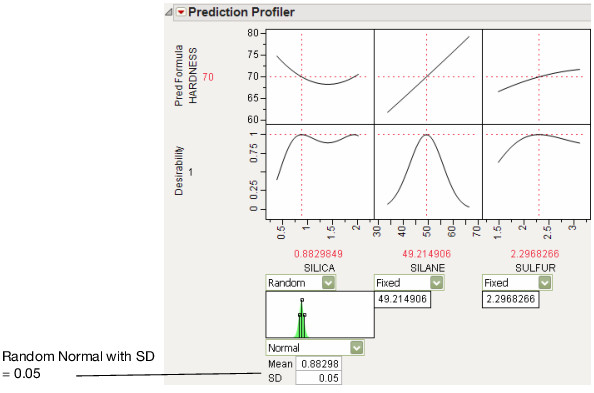3. Click Simulate.

4. Click the Simulate to Table gray triangle and then click the Make Table button.

5. Rename the Pred Formula HARDNESS column to Without Noise Factor.

6. In the Profiler with the noise factor, click the Prediction Profiler red triangle and select Simulator.

7. Assign SILICA to have a random Normal distribution with a standard deviation of 0.05. Use the default setting for the mean.

8. Click Simulate.

9. Click the Simulate to Table gray triangle and then click the Make Table button.

Completing these steps for both the original and noise-factor-optimal simulations results in two similar data tables, one for each simulation. We want to compare the distributions of the predicted hardness from the two simulations. To facilitate the comparison we put the two prediction columns in a single data table.

1. Select the Pred Formula HARDNESS column in the current simulation table and select Edit > Copy With Full Precision.

2. In the other current simulation table, select Cols > New Columns.

3. Type With Noise Factor next to Column Name and click OK.

4. Select the With Noise Factor column and select Edit > Paste.

5. Select Analyze > Distribution.

6. Select With Noise Factor and Without Noise Factor and click Y, Columns.

7. Click OK.

8. Click the Distribution red triangle and select Uniform Scaling.

Figure 3.24 Comparison of Distributions with and without Noise Factors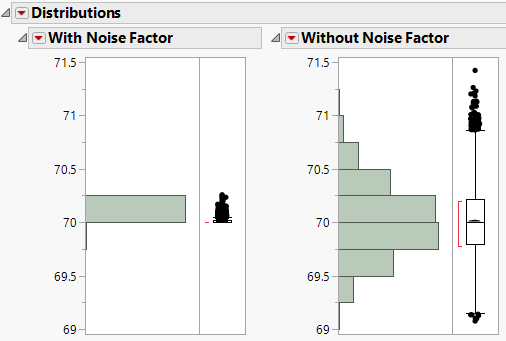The histograms show that there is much more variation in predicted Hardness when the noise factor was not included in the analysis.

It is also interesting to note the shape of the histogram when the noise factor was included. In the comparison histograms above, note that the With Noise Factor distribution has data trailing off in only one direction.The skewness in the With Noise Factor is clear if you deselect Uniform Scaling from the Distributions red triangle menu. The predictions are skewed because Hardness is at a minimum with respect to SILICA, as shown in Figure 3.25. Therefore, variation in SILICA can make only HARDNESS increase. When the non-robust solution is used, the variation could be transmitted either way.

Figure 3.25 Prediction Profiler Showing the Minima of HARDNESS by SILICA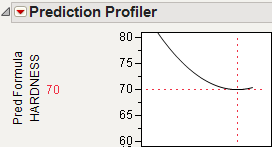Want more information? Have questions? Get answers in the JMP User Community (community.jmp.com).